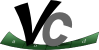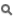Vc  1.1.0 SIMD Vector Classes for C++Vectors

Detailed Description

The vector classes abstract the SIMD registers and their according instructions into types that feel very familiar to C++ developers.

Note that the documented types Vc::float_v, Vc::double_v, Vc::int_v, Vc::uint_v, Vc::short_v, and Vc::ushort_v are actually typedefs of the Vc::Vector<T> class:

namespace Vc {
template<typename T> class Vector;
typedef Vector<double> double_v;
typedef Vector<float> float_v;
// ...
}
Some general information on using the vector classes:

Generally you can always mix scalar values with vectors as Vc will automatically broadcast the scalar to a vector and then execute a vector operation. But, in order to ensure that implicit type conversions only happen as defined by the C standard, there is only a very strict implicit scalar to vector constructor:

int_v a = 1; // good: int_v(int)
uint_v b = 1u; // good: uint_v(unsigned int)
uint_v c = 1; // does not compile: uint_v(int)
float_v d = 1; // does not compile: float_v(int)
float_v e = 1.; // does not compile: float_v(double)
float_v f = 1.f; // good: float_v(float)

The following ways of initializing a vector are not allowed:

int_v v(3, 2, 8, 0); // constructor does not exist because it is not portable
v = 3; v = 2; v = 8; v = 0; // do not hardcode the number of entries!
// You can not know whether somebody will compile with %Vc Scalar where int_v::Size == 1

Instead, if really necessary you can do:

for (int i = 0; i < int_v::Size; ++i) {
v[i] = f(i);
}
// which is equivalent to:
v.fill(f);
// or:

Classes

class  Vector< T, Abi >
The main vector class for expressing data parallelism. More...

Functions

template<typename T , typename Abi >
std::ostream & operator<< (std::ostream &out, const Vc::Vector< T, Abi > &v)
Prints the contents of a vector into a stream object. More...

template<typename V , typename M , typename A >
void deinterleave (V *a, V *b, const M *memory, A align)

Variables

constexpr std::size_t VectorAlignment = alignof(VectorAlignedBase)
Specifies the most conservative memory alignment necessary for Vector<T> objects with default VectorAbi. More...

constexpr std::size_t MemoryAlignment = alignof(MemoryAlignedBase)
Specifies the most conservative memory alignment necessary for aligned loads and stores of Vector types. More...

Vector Type Aliases

using double_v = Vector< double >
vector of double precision

using float_v = Vector< float >
vector of single precision

using int_v = Vector< int >
vector of signed integers

using uint_v = Vector< uint >
vector of unsigned integers

using short_v = Vector< short >
vector of signed short integers

using ushort_v = Vector< ushort >
vector of unsigned short integers

using llong_v = Vector< llong >

using ullong_v = Vector< ullong >

using long_v = Vector< long >

using ulong_v = Vector< ulong >

using schar_v = Vector< schar >

using uchar_v = Vector< uchar >

Function Documentation

 std::ostream& Vc::operator<< ( std::ostream & out, const Vc::Vector< T, Abi > & v )
inline

Prints the contents of a vector into a stream object.

std::cout << v << std::endl;

will output (with SSE):

[0, 1, 2, 3]
Parameters
 out Any standard C++ ostream object. For example std::cout or a std::stringstream object. v Any Vc::Vector object.
Returns
The ostream object: to chain multiple stream operations.
Note
With the GNU standard library this function will check whether the output stream is a tty in which case it colorizes the output.

Definition at line 118 of file IO.

 void Vc::deinterleave ( V * a, V * b, const M * memory, A align )
inline
Deprecated:
Turn to InterleavedMemoryWrapper for a more flexible and complete solution.

Loads two vectors of values from an interleaved array.

Parameters
 a,b The vectors to load the values from memory into. memory The memory location where to read the next 2 * V::Size values from align Either pass Vc::Aligned or Vc::Unaligned. It defaults to Vc::Aligned if nothing is specified.

If you store your data as

struct { float x, y; } m;

then the deinterleave function allows you to read Size concurrent x and y values like this:

This code will load m, m, m, ... into x and m, m, m, ... into y.

The deinterleave function supports the following type combinations:

V \  M | float | double | ushort | short | uint | int
=========|=======|========|========|=======|======|=====
float_v |   X   |        |    X   |   X   |      |
---------|-------|--------|--------|-------|------|-----
double_v |       |    X   |        |       |      |
---------|-------|--------|--------|-------|------|-----
int_v |       |        |        |   X   |      |  X
---------|-------|--------|--------|-------|------|-----
uint_v |       |        |    X   |       |   X  |
---------|-------|--------|--------|-------|------|-----
short_v |       |        |        |   X   |      |
---------|-------|--------|--------|-------|------|-----
ushort_v |       |        |    X   |       |      |

Definition at line 77 of file deinterleave.h.

Variable Documentation

 constexpr std::size_t VectorAlignment = alignof(VectorAlignedBase)

Specifies the most conservative memory alignment necessary for Vector<T> objects with default VectorAbi.

Use this value e.g. with an alignas expression or when allocating aligned memory dynamically (Vc::malloc).

Vc::MemoryAlignment
Vc::VectorAlignedBase

Definition at line 208 of file vector.h.

 constexpr std::size_t MemoryAlignment = alignof(MemoryAlignedBase)

Specifies the most conservative memory alignment necessary for aligned loads and stores of Vector types.

Use this value e.g. with an alignas expression or when allocating aligned memory dynamically (Vc::malloc).